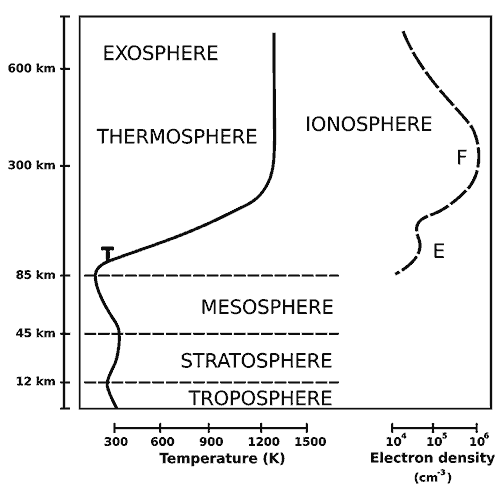# How Earth’s Atmosphere Leak into Space?We all know that our earth’s atmosphere is more or less in a state of hydrostatic equilibrium. Which means, the force due to the pressure gradient in the atmosphere is peacefully balanced by the earth’s gravitational force. Because of this, we have atmospheric layers with heavier elements at the bottom and lighter elements at the top, with a balanced pressure. If gravity is absent, the pressure gradient force will simply leak out our entire atmosphere into the outer space. Lucky for us, we have the right amount of gravity and mostly a non-leaky, good atmosphere that sustains life. However, still a few gases escapes and leaks into the outer space, which is nearly a vacuum. In this edition, we are going to explore why a few gases escape earth’s atmosphere and how they leak into the outer space.

## Turbopause

Our atmosphere consists a few other layers within the primary layers. For this ‘leaky’ analysis, you should know about the turbopause layer, which is found approximately at an altitude of 100 km. Below this turbopause layer is the homosphere where the turbulent atmospheric events (a cyclone) mix the gases and form a homogenous chemical composition. This includes our troposphere, stratosphere, mesosphere and the lower most thermosphere layer close to the mesosphere.

Above that point, lies the heterosphere where the atoms and molecules are free to move for a long path (1 to 10 km) without colliding with any nearby atoms or molecules. Now, molecular weight comes into play and stratification happens. Heavier elements settle at the bottom and the lighter elements like hydrogen and helium gets to the top layer of the thermosphere.T – Turbopause

## Thermosphere and molecular kinetic energy

The average molecular kinetic energy can be related to the temperature with an equation from the kinetic theory (assuming the molecules as point masses) and ideal gas law as shown below.

$$KE_{avg} = \frac{1}{2} mv^2 = \frac{3}{2}kT$$

This tells us that the temperature of the molecule is directly proportional to the kinetic energy of a molecule. In a layer like thermosphere, where the temperature can get as hot as 500 °C to 2500 °C, the molecule attains higher kinetic energy. This higher kinetic energy and relatively lower density of gases in the thermosphere creates a viable environment for the lighter elements to attain the escape velocity of the earth. Indeed, you can compare the average velocity of hydrogen at a temperature, say 1000 °C, with the escape velocity of the earth—11,190 m/s.

From the above equation, we could deduce a relation between the velocity and kinetic energy.

$$\large v_{rms} = \Large\sqrt{\frac{2E_k}{m}}$$

Ek is the kinetic energy of the molecule, m is the mass and vrms is the average velocity.

Mass of a hydrogen molecule H2 is 2.01588 g/mol.

Now let’s find the average kinetic energy of the hydrogen molecule at 1000 °C (1273.15 K) using the first equation.

$$KE = \frac{3}{2} \times 1.3807\times10^{_{-23}} J.K^{-1} \times 1273.15K$$

$$KE = 2.6367\times10^{_{-20}} Joules$$

When we plug this into the second equation, we will get the average velocity (speed).

$$\large v_{rms} = \large\sqrt{\frac{2 \times 2.6367\times10^{_{-20}} J}{2.01588 g}}$$

After some unit conversions, we get the average velocity of a hydrogen molecule at 1000 °C is 1,61,773 m/s. Which is much higher than that of the escape velocity of the earth—11,190 m/s.

Therefore, it is obvious that at such high temperature, few lighter molecules like hydrogen and helium move at these higher velocities and leak out to the outer space. In addition, when they collide, which is so rare, they transfer the energy to a different atom or a molecule. Although, this leakage is so minimum that we lose only a few grams per second.

## Solar winds and the magnetosphere

The second factor that knocks off the gases out to the space is the solar storm. Although we have our protective magnetosphere, the high-energy particles find its way around the earth and sometimes when a few penetrate the magnetosphere, they collide with the molecules and push them off the atmosphere. As the ions tend to align to the direction of the magnetic field, a few ions sometimes are ejected to the outer space. An interesting data observed by a group of satellites called Cluster, shows that at the poles, the earth’s magnetic field is shooting off some oxygen ions. This could also happen at the other elongated end of the magnetosphere, where ions sometimes collide, neutralize themselves, and bid adieu to our earth.

Though planet earth is leaking a few grams of gases a second, it is a very negligible when compared to the total amount. However, it is evident that in our far future, when our sun becomes a red giant, we might lose significant amount of gas including oxygen. For now, breathe well and live well.

This post was first published on October 16, 2015.Karthikeyan KC

Aeronautical engineer, dev, science fiction author, gamer, and an explorer. I am the creator of Geekswipe. I love writing about physics, aerospace, astronomy, and python. I created Swyde. Currently working on Arclind Mindspace.

### 1 Response

1.Jerry

I recently read an article where an experimental Cube Sat was launched with water onboard to be used as fuel. Electrolysis is being used to split off hydrogen and oxygen to be used as fuel to boost the satellites orbit. I wonder if it would be possible for a satellite to collect sufficient Oxygen and Hydrogen in the Thermosphere so that they could be combined to make water and stored onboard as fuel and then use electrolysis to split the water back to Oxygen and Hydrogen so that it could be used as fuel.